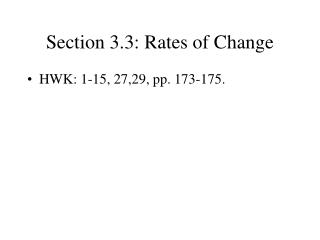DownloadDownload PresentationSection 3.3: Rates of Change

# Section 3.3: Rates of Change

Download Presentation## Section 3.3: Rates of Change

- - - - - - - - - - - - - - - - - - - - - - - - - - - E N D - - - - - - - - - - - - - - - - - - - - - - - - - - -
##### Presentation Transcript

1. Section 3.3: Rates of Change • HWK: 1-15, 27,29, pp. 173-175.

2. We will be dealing with two main concepts: • Average Rate of Change • Instantaneous Rate of Change

3. Average rate of change • Given a function y = f(x) and two values of x (say, a and b), the average rate of change is the quotient (f(b) – f(a))/b-a • That quantity is also sometimes called the difference quotient.

4. Examples: • Average Speed. • Slope of a secant line.

5. Instantaneous Rate of Change • The instantaneous rate of change at x = a is the expected value of the difference quotient (f(a+h) – f(a))/h as h goes to zero.

6. Examples • Instantaneous Velocity • Slope of the tangent line.

7. Examples • Example 3 (page 170) • Example 4 (page 171)